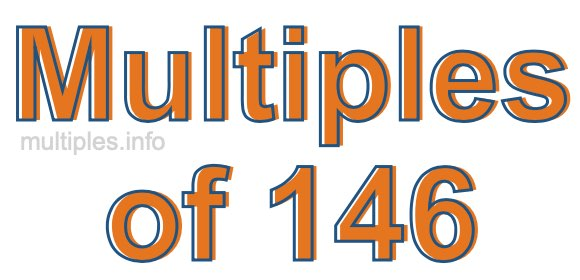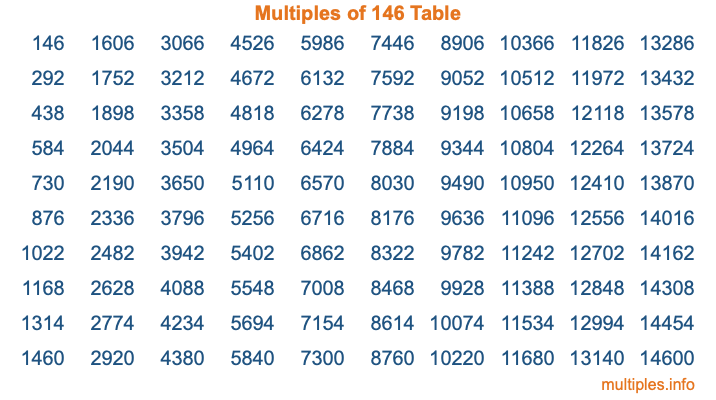Multiples of 146Welcome to the Multiples of 146 page. Here we will first teach you everything you will ever need to know about the multiples of 146, and then give you a study guide summary of everything we taught you to make sure you remember it all. Use this page to look up facts and learn information about the multiples of 146. This page will make you a multiples of one hundred forty-six expert!

Definition of Multiples of 146
Multiples of 146 are all the numbers that when divided by 146 equal an integer. Each of the multiples of 146 are called a multiple. A multiple of 146 is created by multiplying 146 by an integer.

Therefore, to create a list of multiples of 146, you start with 1 multiplied by 146, then 2 multiplied by 146, then 3 multiplied by 146, and so on for as long as you want. Thus, the list of the first five multiples of 146 is 146, 292, 438, 584, and 730. To see a larger list of multiples of 146, see the printable image of Multiples of 146 further down on this page. We also have a category where you can choose any nth multiple of 146.

Multiples of 146 Checker
The Multiples of 146 Checker below checks to see if any number of your choice is a multiple of 146. In other words, it checks to see if there is any number (integer) that when multiplied by 146 will equal your number. To do that, we divide your number by 146. If the the quotient is an integer, then your number is a multiple of 146.

Is  a multiple of 146?

Least Common Multiple of 146 and ...
A Least Common Multiple (LCM) is the lowest multiple that two or more numbers have in common. This is also called the smallest common multiple or lowest common multiple and is useful to know when you are adding our subtracting fractions. Enter one or more numbers below (146 is already entered) to find the LCM.

Check out our LCM Calculator if you need more details about the Least Common Multiple or if you need the LCM for different numbers for adding and subtraction fractions.

nth Multiple of 146
As we stated above, 146 is the first multiple of 146, 292 is the second multiple of 146, 438 is the third multiple of 146, and so on. Enter a number below to find the nth multiple of 146.

th multiple of 146

Multiples of 146 vs Factors of 146
146 is a multiple of 146 and a factor of 146, but that is where the similarities end. All postive multiples of 146 are 146 or greater than 146. All positive factors of 146 are 146 or less than 146.

Below is the beginning list of multiples of 146 and the factors of 146 so you can compare:

Multiples of 146: 146, 292, 438, 584, 730, etc.

Factors of 146: 1, 2, 73, 146

As you can see, the multiples of 146 are all the numbers that you can divide by 146 to get a whole number. The factors of 146, on the other hand, are all the whole numbers that you can multiply by another whole number to get 146.

It's also interesting to note that if a number (x) is a factor of 146, then 146 will also be a multiple of that number (x).

Multiples of 146 vs Divisors of 146
The divisors of 146 are all the integers that 146 can be divided by evenly. Below is a list of the divisors of 146.

Divisors of 146: 1, 2, 73, 146

The interesting thing to note here is that if you take any multiple of 146 and divide it by a divisor of 146, you will see that the quotient is an integer.

Multiples of 146 Table
Below is an image of the first 100 multiples of 146 in a table. The table is in chronological order, column by column. The first column has the first ten multiples of 146, the second column has the next ten multiples of 146, and so on.The Multiples of 146 Table is also referred to as the 146 Times Table or Times Table of 146. You are welcome to print out our table for your studies.

Negative Multiples of 146
Although not often discussed or needed in math, it is worth mentioning that you can make a list of negative multiples of 146 by multiplying 146 by -1, then by -2, then by -3, and so on, to get the following list of negative multiples of 146:

-146, -292, -438, -584, -730, etc.

Multiples of 146 Summary
Below is a summary of important Multiples of 146 facts that we have discussed on this page. To retain the knowledge on this page, we recommend that you read through the summary and explain to yourself or a study partner why they hold true.

There are an infinite number of multiples of 146.

A multiple of 146 divided by 146 will equal a whole number.

146 divided by a factor of 146 equals a divisor of 146.

The nth multiple of 146 is n times 146.

The largest factor of 146 is equal to the first positive multiple of 146.

146 is a multiple of every factor of 146.

146 is a multiple of 146.

A multiple of 146 divided by a divisor of 146 equals an integer.

146 divided by a divisor of 146 equals a factor of 146.

Any integer times 146 will equal a multiple of 146.

Multiples of a Number
Here you can get the multiples of another number, all with the same attention to detail as we did for multiples of 146 on this page.

Multiples of
Multiples of 147
Did you find our page about multiples of one hundred forty-six educational? Do you want more knowledge? Check out the multiples of the next number on our list!